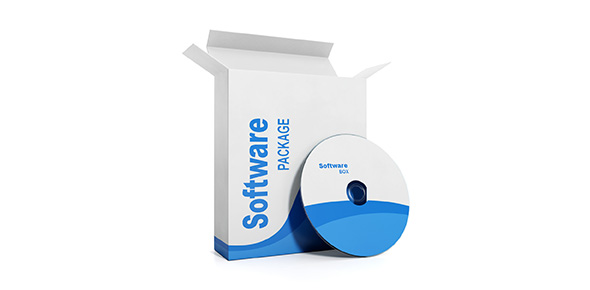# [s1g1] Python Typecasting Cw

17 Questions | Total Attempts: 45SettingsCreate your own QuizRead the questions very carefully and solve them in PyCharm

• 1.
Givenvar1="5.0"write python code to modify and typecast num such thatprint(type(var1)) outputs as  <class 'int'>
• 2.
Write the output for the following lines of code var1=str(34.5+2)+str(34.5+2) print(var1)
• 3.
A="12.5" #line 1 b="13.5" #line2 c=??? <-------- line 3 print(c) In the above program complete line 3 with instruction using only typecasting command i.e int(), str(),float() and mathematical operators such that the output is 26
• 4.
Write the output for the following lines of code var1=5 print(float(var1))
• 5.
Write the output for the following lines of code var1="5" print(float(var1))
• 6.
Write the output for the following lines of code var1="5" var2="2" print(float(var1/var2))
• 7.
Write the output for the following lines of code var1="5/2" print(int(var1))
• 8.
Write the output for the following lines of code var1=str(int("2"))+"ab" print(var1)
• 9.
Write the output for the following lines of code var1=str(34+2)+str(34+2) print(var1)
• 10.
Write the output for the following lines of code var1=str(34+2)+int(34+2) print(var1)
• 11.
Write the output for the following lines of code var1=int("-20"+str(20)) print(var1)
• 12.
Write the output for the following lines of code var1=int("12")//float(2) print(var1)
• 13.
A="12.5" #line 1 b="13.5" #line2 c=??? <-------- line 3 print(c) In the above program complete line 3 with instruction using only typecasting command i.e int(), str(),float() and mathematical operators such that the output is 26.0
• 14.
a="12.5" #line 1 b="13.5" #line2 c="hello" #line 3 d=??? <-------- line 4 print(d) In the above program complete line 4 with instruction using only typecasting command i.e int(), str(),float() and mathematical operators such that the output is 26.0hello
• 15.
A=25 #line 1 b=30 #line 2 c="hello" #line 3 d=??? <-------- line 4 print(d)In the above program complete line 4 using the variables a,b,c with typecasting command i.e int(), str(),float() and mathematical operators such that the output is 2530hello
• 16.
A=0.25 #line 1 b=0.75 #line2 c="c" #line 3 d=??? <-------- line 4 print(d) In the above program replace line 2 with instruction using typecasting command i.e int(), str(),float() such that the output is 1c
• 17.
a=12 #line 1 b=12 #line2 c="/" #line 3 d=??? <-------- line 4 print(d) In the above program replace line 4 with instruction using typecasting command i.e int(), str(),float() and mathematical operators such that the output is 12/12Back to top
×

Wait!
Here's an interesting quiz for you.